Cross Multiplication Method

Chapter 3 Class 10 Pair of Linear Equations in Two Variables
Serial order wise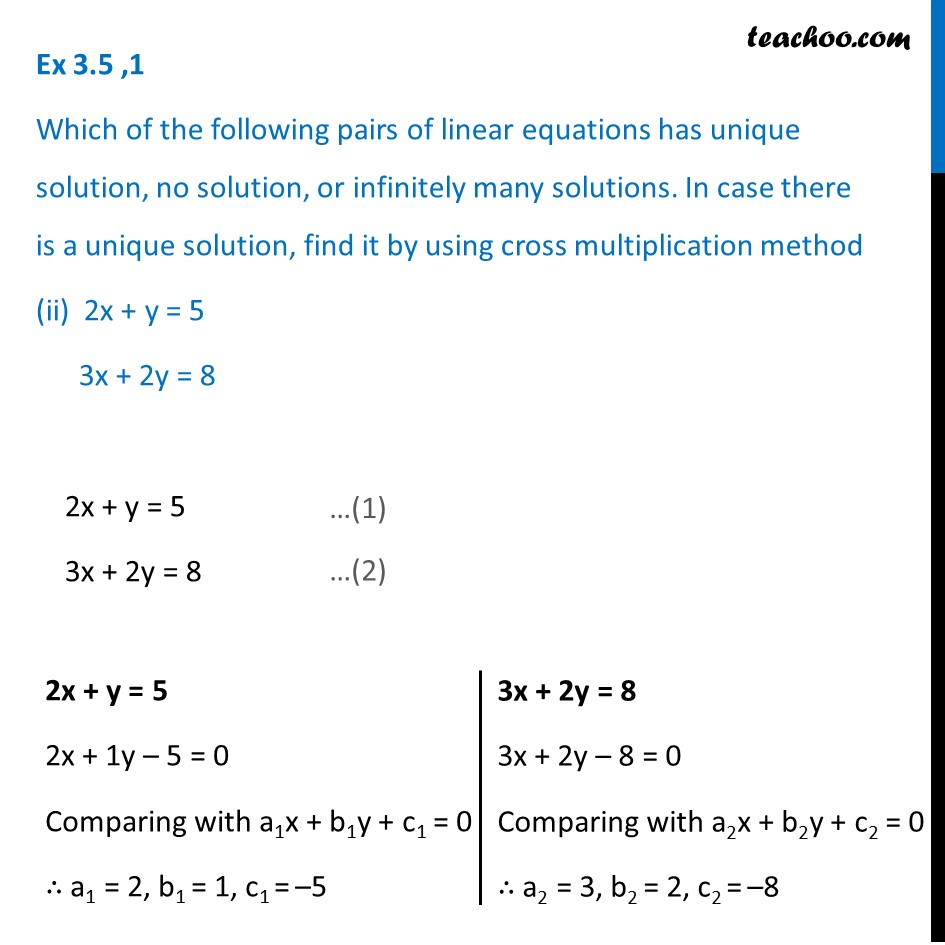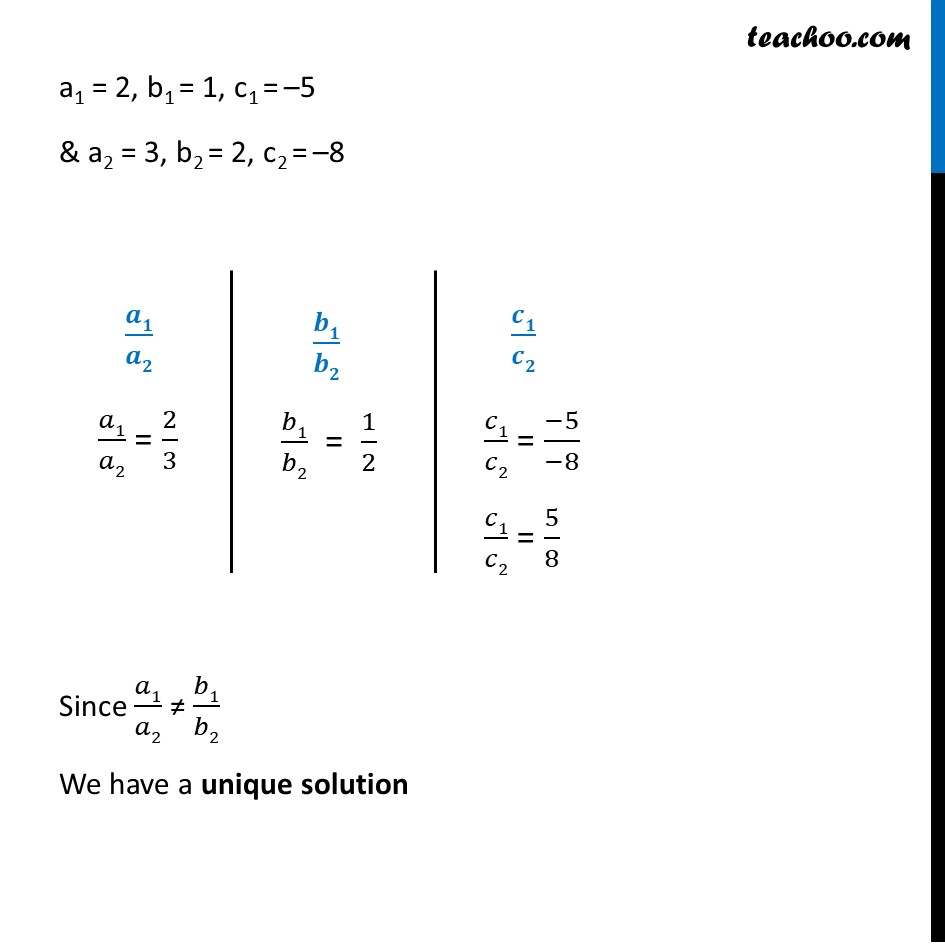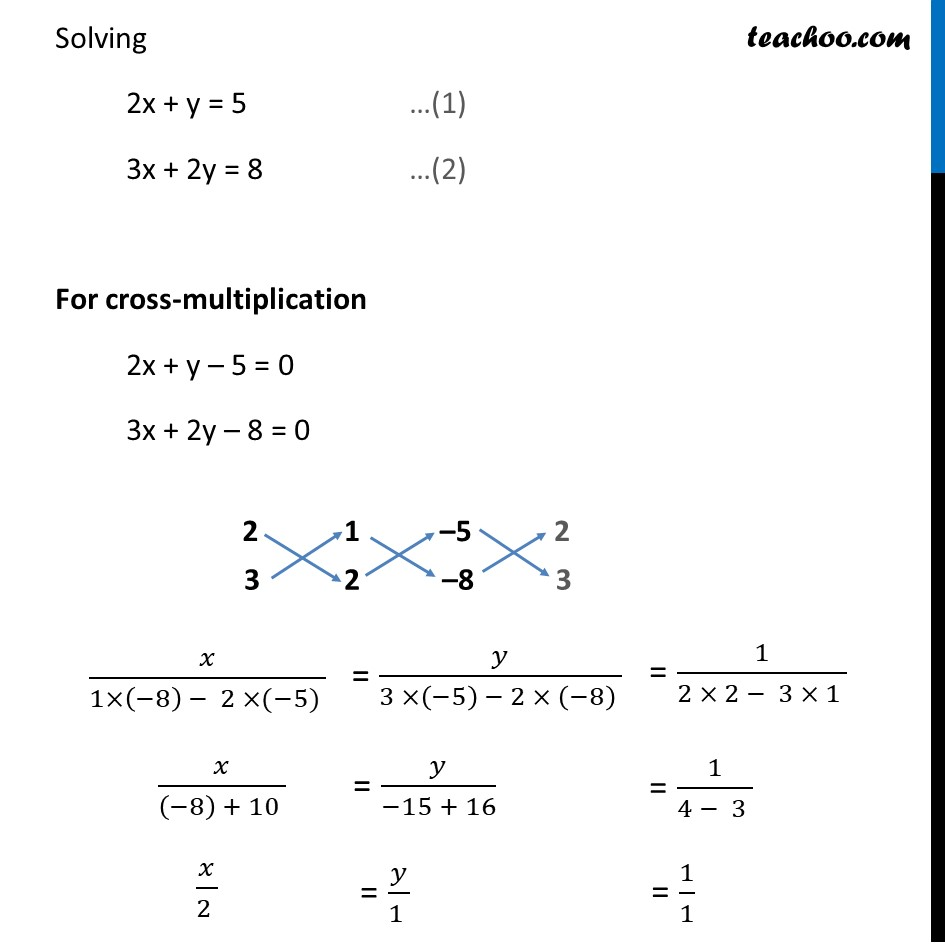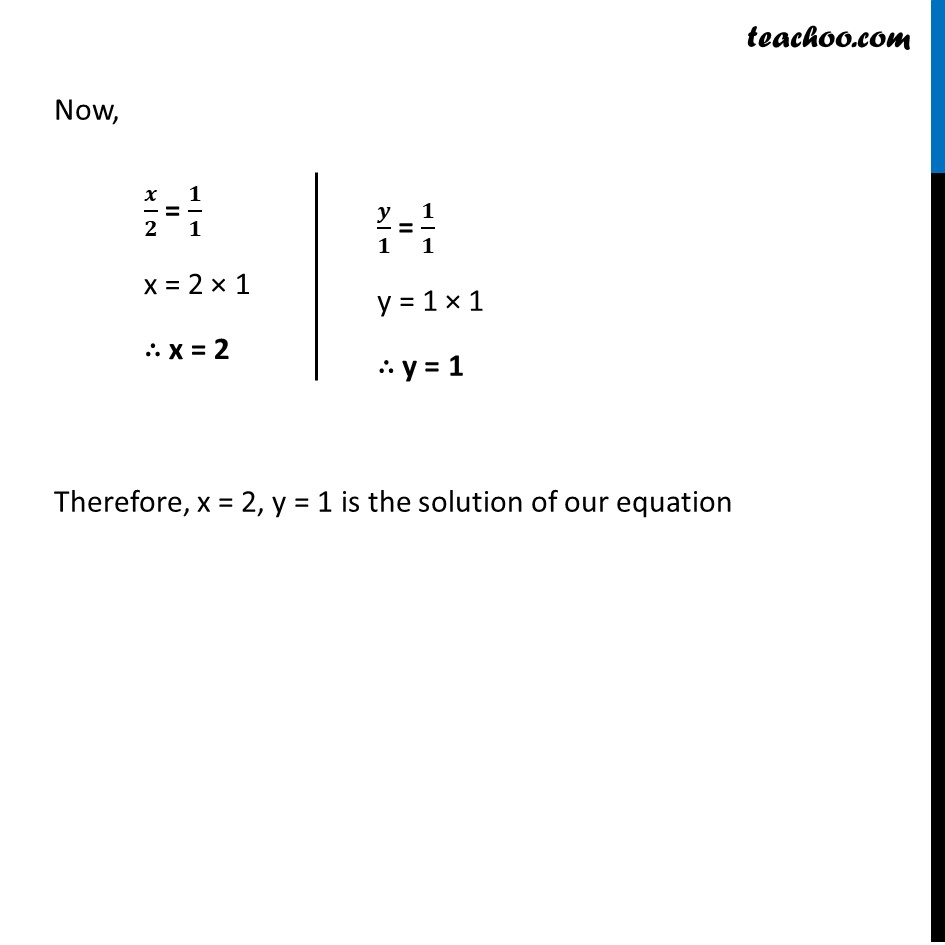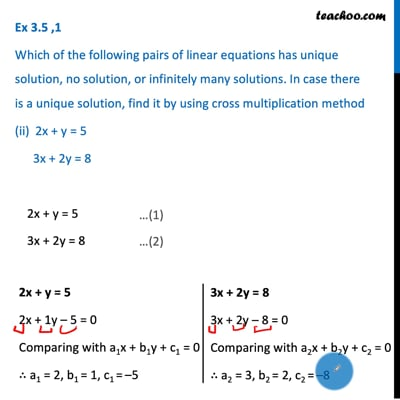This video is only available for Teachoo black users

Learn in your speed, with individual attention - Teachoo Maths 1-on-1 Class

### Transcript

Ex 3.5 ,1 Which of the following pairs of linear equations has unique solution, no solution, or infinitely many solutions. In case there is a unique solution, find it by using cross multiplication method (ii) 2x + y = 5 3x + 2y = 8 2x + y = 5 3x + 2y = 8 2x + y = 5 2x + 1y – 5 = 0 Comparing with a1x + b1y + c1 = 0 ∴ a1 = 2, b1 = 1, c1 = –5 3x + 2y = 8 3x + 2y – 8 = 0 Comparing with a2x + b2y + c2 = 0 ∴ a2 = 3, b2 = 2, c2 = –8 a1 = 2, b1 = 1, c1 = –5 & a2 = 3, b2 = 2, c2 = –8 𝒂𝟏/𝒂𝟐 𝑎1/𝑎2 = 2/3 𝒃𝟏/𝒃𝟐 𝑏1/𝑏2 = 1/2 𝒄𝟏/𝒄𝟐 𝑐1/𝑐2 = (−5)/(−8) 𝑐1/𝑐2 = 5/8 Since 𝑎1/𝑎2 ≠ 𝑏1/𝑏2 We have a unique solution Solving 2x + y = 5 …(1) 3x + 2y = 8 …(2) For cross-multiplication 2x + y – 5 = 0 3x + 2y – 8 = 0 𝑥/(1×(−8) − 2 ×(−5) ) 𝑥/((−8) + 10 ) 𝑥/(2 ) = 𝑦/(3 ×(−5) − 2 × (−8) ) = 𝑦/(−15 + 16) = 𝑦/(1 ) = 1/(2 × 2 − 3 × 1 ) = 1/(4 − 3 ) = 1/1 Now, 𝒙/𝟐 = 𝟏/𝟏 x = 2 × 1 ∴ x = 2 𝒚/𝟏 = 𝟏/𝟏 y = 1 × 1 ∴ y = 1 Therefore, x = 2, y = 1 is the solution of our equation